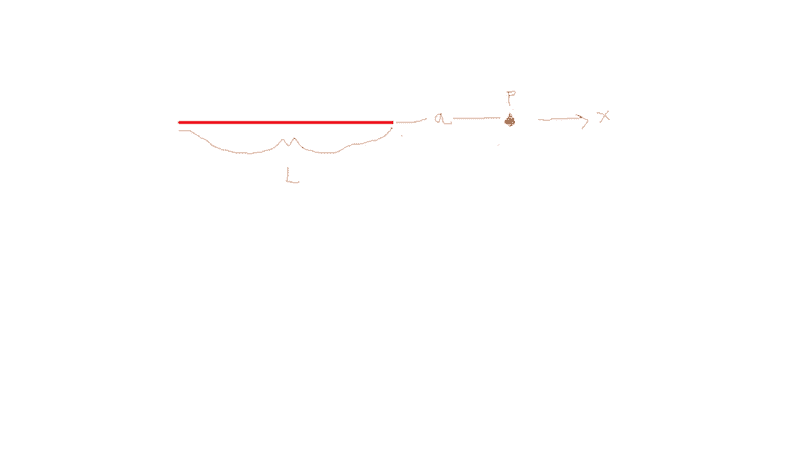Electric Field -- Rod from far away

Gold Member

Homework Statement

Theres a rod and has ##a## lenght ##L## and we want to calculaate the electric field far from rod like ##L<<a##

The Attempt at a Solution

I found the equation for a normal case and it was ##\frac{1}{4\pi e_0}\frac{2Q}{L^2}\left(ln\left(\frac{a}{L+a}\right)+\frac{L}{a}\right)## .Itn non-uniform thats why theres ##2## anyway in the ##L<<a## case it will be just ##\frac{1}{4\pi e_0}\frac{2Q}{L^2}## .
You might ask you did so far how you cant do that well I am not sure about the ##\frac {L} {a}## term it seem like it will be ##\frac {1} {a}## but in that case the units will not match so it must be 0.When something like this happens we will think ##a→∞## ?

haruspex
Homework Helper
Gold Member
well I am not sure about the ##\frac {L} {a}## term it seem like it will be ##\frac {1} {a}##
I don't know why you think it would be like 1/a. As you say, it will tend to zero as a tends to infinity, but the same is true of the log term, leaving you with zero overall. Of course, that is trivially true, but we need the first nonzero term in the approximation.
So you need to start by expanding the ln as a power series.

Gold Member
Of course, that is trivially true, but we need the first nonzero term in the approximation.
So you need to start by expanding the ln as a power series.
It was used as a hint in our homework but I didnt give too much attantion cause ##ln\left(\frac{a}{L+a}\right)## would be just zero.Why do I need to write in as power series..?
In our homework as a hint it says ##ln(1+ε)=ε-\frac {e^2} {2}+\frac {e^3} {2}..## so ##ln\left(\frac{a}{L+a}\right)## can be written as ##-ln\left(\frac{L+a}{a}\right)##
and that is ##-ln\left(\frac{L}{a}+1\right)## so ##ε=\frac {L} {a}##
so simply
##-ln\left(\frac{L}{a}+1\right)+\frac {L} {a}=-ε+ε=0## (cause ##\frac {L} {a}=ε##)

Gold Member
Should ı write like this ?

gneill
Mentor

Homework Statement

Theres a rod and has ##a## lenght ##L## and we want to calculaate the electric field far from rod like ##L<<a##
Presumably the rod is charged in some way? Uniformly?
How is the point at distance ##a## positioned with respect to the rod? For clarity, suppose that the rod is lying along the y-axis with its center at the origin. Is the point ##a## located somewhere along the x-axis, or perhaps the y-axis?

Gold Member
Presumably the rod is charged in some way? Uniformly?
How is the point at distance ##a## positioned with respect to the rod? For clarity, suppose that the rod is lying along the y-axis with its center at the origin. Is the point ##a## located somewhere along the x-axis, or perhaps the y-axis?
I wrote the question so silly way sorry for that.Charge distrubiton is not uniform and its like λ(x)=bx (https://www.physicsforums.com/threads/electric-field-dimension-analysis.906014/ this thread also the first 2 parts of my question)
Here a picture to make things clearI choose the left end of the rod is origin.

Gold Member
its positivly charged

haruspex
Homework Helper
Gold Member
It was used as a hint in our homework but I didnt give too much attantion cause ##ln\left(\frac{a}{L+a}\right)## would be just zero.Why do I need to write in as power series..?
In our homework as a hint it says ##ln(1+ε)=ε-\frac {e^2} {2}+\frac {e^3} {2}..## so ##ln\left(\frac{a}{L+a}\right)## can be written as ##-ln\left(\frac{L+a}{a}\right)##
and that is ##-ln\left(\frac{L}{a}+1\right)## so ##ε=\frac {L} {a}##
so simply
##-ln\left(\frac{L}{a}+1\right)+\frac {L} {a}=-ε+ε=0## (cause ##\frac {L} {a}=ε##)
That's the right approach, but you need to keep another term from the expansion. This a general rule in problems like this... keep just enough terms that the result is not zero.

Gold Member
That's the right approach, but you need to keep another term from the expansion. This a general rule in problems like this... keep just enough terms that the result is not zero.
Why are leaving another term ? Dont we want it to be a zero ?
So we will write ##-e+\frac {e^2} {2}+e## and ##\frac {e^2} {2}=0## again ? (e means epsilon)

Gold Member
##\frac {ε^2} {2}## will not be zero its ##\frac {L^2} {2a^2}## so my E will be
##\frac {1} {4πε_0} \frac {Q} {a^2}## which thats what we want.
so the other terms would be zero ?

gneill
Mentor
so the other terms would be zero ?
No, the other terms would be considered to be negligible.

Gold Member
No, the other terms would be considered to be negligible.
Oh I see your point ok